# NCERT Solutions for Class 12 Physics Chapter 6 Electromagnetic Induction Hindi

## NCERT Solutions for Class 12 Physics Chapter 6 Electromagnetic Induction Hindi Medium

Download the Class 12 Physics NCERT Solutions in Hindi medium and English medium as well offered by the leading e-learning platform Vedantu. If you are a student of Class 12, you have reached the right platform. The NCERT Solutions for Class 12 Physics in Hindi provided by us are designed in a simple, straightforward language, which are easy to memorise. You will also be able to download the PDF file for NCERT Solutions for Class 12 Physics  in Hindi from our website at absolutely free of cost.

NCERT, which stands for The National Council of Educational Research and Training, is responsible for designing and publishing textbooks for all the classes and subjects. NCERT textbooks covered all the topics and are applicable to the Central Board of Secondary Education (CBSE) and various state boards.

We, at Vedantu, offer free NCERT Solutions in English medium and Hindi medium for all the classes as well. Created by subject matter experts, these NCERT Solutions in Hindi are very helpful to the students of all classes.

Do you need help with your Homework? Are you preparing for Exams?
Study without Internet (Offline)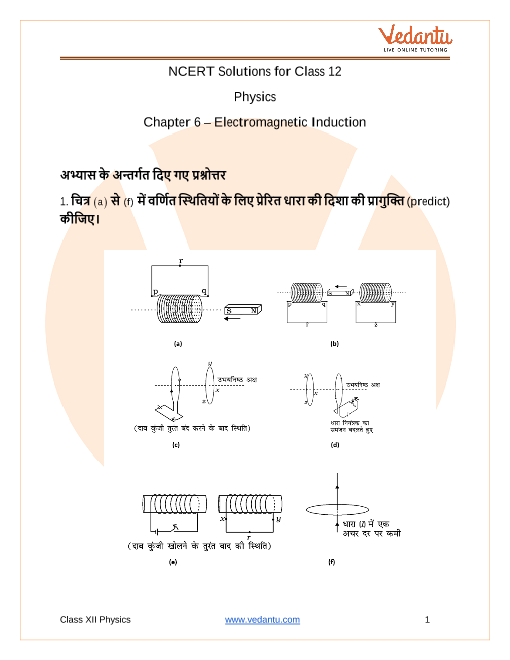## Access NCERT Solutions for Science (Physics) Chapter 6- Electromagnetic Induction

अभ्यास के अन्तर्गत दिए गए प्रश्नोत्तर

1. चित्र $\left( a \right)$ से $(f)$ में वर्णित स्थितियों के लिए प्रेरित धारा की दिशा की प्रागुक्ति (predict) कीजिए।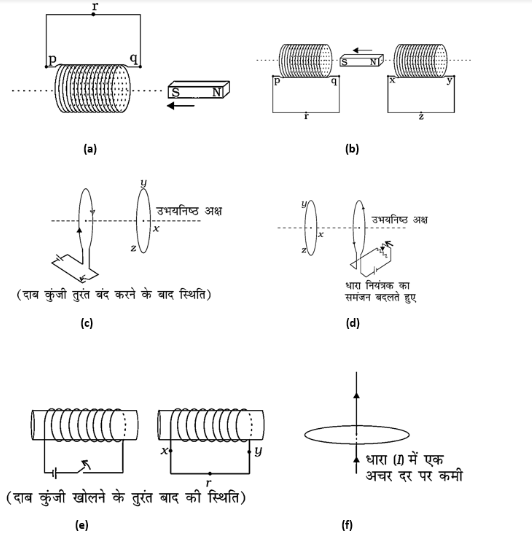उत्तर-
(a) चुम्बक के $S$ ध्रुव को कुण्डली की ओर ले जाया जा रहा है, अतः लेन्ज के नियम के अनुसार कुण्डली का यह पक्ष भी है $S$ ध्रुव ऐसा होना चाहिए कि वह चुंबक की गति का विरोध करे (पारस्परिक प्रतिकर्षण द्वारा) ताकि कुंडली में प्रेरित धारा दक्षिणावर्त दिशा में हो अर्थात। $qrpq$ दिशा में बहेगी।

(b) लेन्ज के नियम के अनुसार चुम्बक की गति का विरोध करने के लिए चुम्बक के बायीं ओर कुण्डली का ध्रुव $S$ की ओर वाला सिरा $S$ बनना चाहिए तथा दायीं ओर की कुण्डली का चुम्बक में $N$ ध्रुव की ओर वाला सिरा भी $S$ ध्रुव ही बनना चाहिए ताकि ध्रुव $S$ पर प्रतिकर्षण तथा $N$ लेकिन आकर्षण का बल था। अतः बायें हाथ की कुण्डली में धारा दक्षिणावर्त दिशा में है।(अर्थात् $prqp$ दिशा में), तथा दायीं ओर की कुण्डली में धारा $yzxy$ दिशा में प्रेरित होनी चाहिए।

(c) प्रेशर की को तुरंत बंद करने पर बायें हाथ की कुण्डली में धारा शून्य से बढ़ जाएगी, इसलिए दायें हाथ की कुण्डली में प्रेरित धारा बायें हाथ की कुण्डली में धारा की विपरीत दिशा में होती है (अर्थात वामावर्त दिशा में) .$yzx$ में होनी चाहिए।

(d) चित्र से स्पष्ट है कि करंट कंट्रोलर द्वारा रेजिस्टेंस को कम किया जा रहा है, यानी दाईं ओर कॉइल में करंट बढ़ेगा, जिसकी दिशा वामावर्त है। इसलिए, लेन्ज़ के नियम के अनुसार, बाएं हाथ की कुंडली में प्रेरित धारा मुख्य धारा के विपरीत होनी चाहिए, अर्थात। $zyx$ दिशा में।

(e) प्राथमिक कुण्डली में दाब कुंजी खोलने के तुरन्त बाद धारा कम हो जाएगी। अतः द्वितीयक कुण्डली में धारा की दिशा प्राथमिक की मुख्य धारा की दिशा में होनी चाहिए, अर्थात्।$xry$ दिशा में।

(f) कोई प्रेरित धारा नहीं है क्योंकि बल की रेखाएं लूप के तल में स्थित होंगी और फ्लक्स नहीं बदलेगा। चूंकि बल की रेखाएं लूप को भी नहीं काटेंगी।

2. चित्र  में वर्णित स्थितियों के लिए लेंज के नियम का उपयोग करते हुए प्रेरित विद्युत धारा की दिशा ज्ञात कीजिए।
(a) जब अनियमित आकार का तार वृत्ताकार लूप में बदल रहा हो;
(b) जब एक वृत्ताकार लूप एक सीधे तार में विरूपित किया जा रहा हो।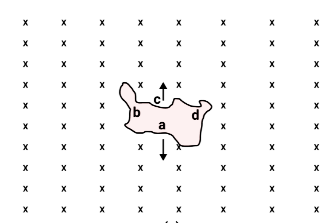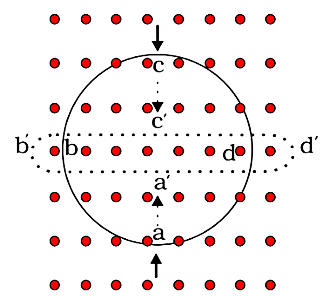उत्तर-
(a) क्रॉस $(x)$ द्वारा एक ऐसे चुम्बकीय-क्षेत्र को प्रदर्शित किया गया है जिसकी दिशा कागज के तल के लम्बवत् भीतर की ओर है अनियमित आकार के लूप को वृत्तीय रूप में खींचने पर इससे गुजरने वाला फ्लक्स बढ़ेगा। अतः लूप में प्रेरित धारा इस प्रकार की होगी कि वह निम्नगामी फ्लक्स को बढ़ने से रोकेगी। प्रेरित धारी कागज के तल के लम्बवत् ऊपर की ओर चुम्बकीय-क्षेत्र उत्पन्न करेगी। अत: धारा की दिशा $a{\text{ }}d{\text{ }}c{\text{ }}b{\text{ }}a$ मार्ग का अनुसरण करेगी।

(b) चुंबकीय क्षेत्र कागज के तल के लंबवत बाहर की ओर होता है। लूप का आकार बदलने पर, इससे गुजरने वाला ऊपर की ओर प्रवाह कम हो जाएगा। इसलिए, लूप में प्रेरित धारा ऊपर की ओर चुंबकीय क्षेत्र उत्पन्न करेगी।इसके लिए धारा  मार्ग का अनुसरण करेगी।

3. एक लम्बी परिनालिका के इकाई सेंटीमीटर लम्बाई में $15$ फेरे हैं। उसके अन्दर $2.0{\text{cm}}$ का एक छोटा-सा लूप परिनालिका की अक्ष के लम्बवत रखा गया है। यदि परिनालिका में बहने वाली धारा का मान $0.15$ में $2.0{\text{ }}A$ से $40{\text{ }}A$ कर दिया जाए तो धारा परिवर्तन के समय प्रेरित विद्युत वाहक बल कितना होगा?

हल- परिनालिका में फेरों की संख्या N=15, लम्बाई $l = 1{\text{cm}} = 0.01{\text{m}}$

${i_1} = 2.0{\text{A}},{\text{}}{i_2} = 4.0{\text{A}},{\Delta}t = 0.1{\text{s}}$

लूप का क्षेत्रफल $A = 2.0{\text{c}}{{\text{m}}^2} = 2.0 \times {10^{ - 4}}{\text{}}{{\text{m}}^2}$
लूप में प्रेरित विद्युत वाहक बल

$e = n\dfrac{{d\phi }}{{dt}} = nA\dfrac{{dB}}{{dt}}$

जबकि परिनालिका के अक्ष पर क्षेत्रफल में परिवर्तन

${dB}{ = \dfrac{{{\mu _0}N}}{l}\left( {{i_2} - {i_1}} \right)}$

${ = \dfrac{{4\pi \times {{10}^{ - 7}} \times 15}}{{0.01}}\left( {4.0 - 2.0} \right) = 120\pi \times {{10}^{ - 5}}}$

$e{ = \dfrac{{1 \times 2.0 \times {{10}^{ - 4}} \times 120\pi \times {{10}^{ - 5}}}}{{0.01}}}$

${ = 7.54 \times {{10}^{ - 6}}{\text{V}} \approx 7.5 \times {{10}^{ - 6}}{\text{V}}}$

4. एक आयताकार लूप जिसकी भुजाएँ $8 \mathrm{~cm}$ एवं $2 \mathrm{~cm}$ हैं, एक स्थान पर थोड़ा कटा हुआ है। यह लूप अपने तल के अभिलंबवत $0.3 \mathrm{~T}$ के एकसमान चुंबकीय क्षेत्र से बाहर की ओर निकल रहा है। यदि लूप के बाहर निकलने का वेग $1 \mathrm{~cm} \mathrm{~s}^{-1}$ है तो कटे भाग के सिरों पर उत्पन्न विद्युत वाहक बल कितना होगा, जब लूप की गति अभिलंबवत हो (a) लूप की लंबी भुजा के (b) लूप की छोटी भुजा के। प्रत्येक स्थिति में उत्पन्न प्रेरित वोल्टता कितने समय तक टिकेगी?
हल-

(a) चुम्बकीय क्षेत्र B में क्षेत्र के लम्बवत् स्थित क्षेत्रफल $A$ से गुजरने वाला चुम्बकीय फ्लक्स $F = BA$
माना लूप की लम्बाई $l$ व चौड़ाई $b$ और इसके वेग का परिमाण है। जैसे ही लूप को चुंबकीय क्षेत्र से लंबी भुजा के लंबवत ले जाया जाता है, क्षेत्र से जुड़ा क्षेत्र बदल जाता है, जिससे परिवर्तन होता है। फैराडे के नियम के अनुसार, प्रेरित विद्युत वाहक बल का परिमाण

$|e| = \dfrac{{d\Phi }}{{dt}} = \dfrac{d}{{dt}}(BA) = B\left( {\dfrac{{dA}}{{dt}}} \right)$

अब, $\quad \dfrac{{dA}}{{dt}} = lv$

(प्रति सेकण्ड लूप द्वारा घिरा क्षेत्रफल)

$|e| = Blv = 0.3 \times \left( {8 \times {{10}^{ - 2}}} \right) \times \left( {{{10}^{ - 2}}} \right)$

$= 24 \times {10^{ - 4}}$

$= 2.4 \times {10^{ - 4}}$

$= 0.24{\text{mV}}$

प्रेरित विभवान्तर तब तक रहेगा जब तक फ्लक्स परिवर्तित होगा। इस प्रकार, विभवान्तर $|e|$ के बने रहने का समय

$= \dfrac{b}{v} = \dfrac{{2 \times {{10}^{ - 2}}{\text{ }}}}{{{{10}^{ - 2}}/}}$

$= 2{\text{s}}$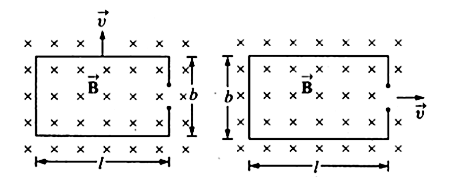(b) लूप की छोटी भुजा के। प्रत्येक स्थिति में उत्पन्न प्रेरित वोल्टता कितने समय तक टिकेगी?

(b) चित्र 6.3(b) से, $\dfrac{{dA}}{{dt}} = bv$

${\left| e \right|}{ = Bbv = 0.3 \times \left( {2 \times {{10}^{ - 2}}} \right) \times {{10}^{ - 2}}}$

${ = 0.6 \times {{10}^{ - 4}}{\text{}}}$

${ = 0.06{\text{}}}$

|e| के बने रहने का समय $= \dfrac{l}{v} = \dfrac{{8 \times {{10}^{ - 2}}{\text{}}}}{{{{10}^{ - 2}}{\text{}}}}=8$s

5. $1.0{\text{m}}$ लम्बी धातु की छड़ उसके एक सिरे से जाने वाले अभिलम्बवत अक्ष के परितः $400{\text{ }}rad - {s^{ - 1}}$ की कोणीय आवृत्ति से घूर्णन कर रही है। छड़ का दूसरा सिरा एक धात्विक वलय से सम्पर्कित है। अक्ष के अनुदिश सभी जगह $0.5{\text{ }}T$ का एकसमान चुम्बकीय-क्षेत्र उपस्थित है। वलय तथा अक्ष के बीच स्थापित विद्युत वाहक बल की गणना कीजिए।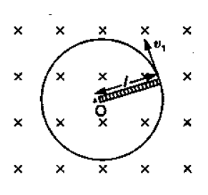हल- दिया है, धात्विक छड़ की लम्बाई $l = 1.0$ मीटर कोणीय आवृत्ति $\omega = 400$ रेडियन/सेकण्ड

चुम्बकीय क्षेत्र $B = 0.5$ टेस्ला

छड़ के एक सिरे का वेग ${v_1} = 0$

छड़ के दूसरे सिरे का वेग ${v_2} = l\omega$

अत: छड़ का औसत रेखीय वेग

$v = \dfrac{{{v_1} + {v_2}}}{2}$ $v = \dfrac{{0 + l\omega }}{2} = \dfrac{{\omega l}}{2}$

अत: केन्द्र $O$ व वलय के बीच प्रेरित वै० वा० बल

$E{\text{ }} = lvB = l \times \dfrac{{\omega l}}{2} \times B = \dfrac{1}{2}\omega B{l^2}$

$= \dfrac{1}{2} \times 400 \times 0.5 \times {(1.0)^2}$

$= 100$V

6. एक वृत्ताकार कुंडली जिसकी त्रिज्या $8.0{\text{ }}cm$ तथा फेरों की संख्या $20$ है अपने ऊर्ध्वाधर व्यास के परितः $50{\text{rad }} s^{-1}$ की कोणीय आवृत्ति से $3.0{\text{ }} \times {10^{ - 2}}T$ के एकसमान चुम्बकीय-क्षेत्र में घूम रही है। कुंडली में उत्पन्न अधिकतम तथा औसत प्रेरित विद्युत वाहक बल का मान ज्ञात कीजिए। यदि कुंडली $10{\text{ }}\Omega$ प्रतिरोध का एक बन्द लूप बनाए तो कुंडली में धारा के अधिकतम मान की गणना कीजिए। जूल ऊष्मन के कारण क्षयित औसत शक्ति की गणना कीजिए। यह शक्ति कहाँ से प्राप्त होती है?
हल-कुण्डली में बद्ध चुम्बकीय फ्लक्स

$\phi = \vec B \cdot \vec A = BA{\text{cos}}\theta = BA{\text{cos}}\omega t\left( {{\text{as}}\theta = \omega t} \right)$

कुण्डली में प्रेरित वै० वा० बल, $\varepsilon = - N\dfrac{{d\phi }}{{dt}}$

$= - N\dfrac{d}{{dt}}\left( {BA{\text{cos}}\omega t} \right) = NBA\omega {\text{sin}}\omega t$

अधिकतम प्रेरित वै० वा० बल, ${\varepsilon _{{\text{max}}}} = NBA\omega = NB\left( {\pi {r^2}} \right)\omega$
यहाँ $N = 20,r = 8.0cm$

$=8.0 \times {10^{ - 2}}$m,

$B{ = 3.0 \times {{10}^{ - 2}}{\text{T}},\omega = 50{s^{ - 1}}}$

${\therefore {\varepsilon _{{\text{max}}}}}{ = 20 \times 3.0 \times {{10}^{ - 2}} \times 3.14 \times {{\left( {8.0 \times {{10}^{ - 2}}} \right)}^2} \times 50}$

${ = 0.603{\mathbf{V}}}$

सत वै० वा० बल, ${\varepsilon _{av}} = NAB\omega {({\text{sin}}\omega t)_{av}} = 0$
(पूरे चक्र के लिए ${\text{sin}}\omega t$ शून्य का औसत मान है।)
अधिकतम प्रेरित धारा, ${I_{{\text{max}}}} = \dfrac{{{\varepsilon _{{\text{max}}}}}}{R} = \dfrac{{0.603}}{{10}} = 0.603{\text{A}}$
जूल ऊष्मा के कारण औसत व्यय शक्ति, ${P_{{\text{max}}}} = {\left( {{I^2}} \right)_{av}}R$

${P_{{\text{max}}}} = \dfrac{{{{\left( {{t^2}} \right)}_{av}}}}{R} = \dfrac{1}{2}\dfrac{{\varepsilon _{{\text{max}}}^2}}{R}$

[क्योंकि पूरे चक्र के लिए ${\text{si}}{{\text{n}}^2}\omega t$ का औसत मान $\dfrac{1}{2}$ होता है। $\therefore \varepsilon_{a v}^{2}=\dfrac{\varepsilon_{\max }^{2}}{2}$

$\therefore {\text{}}{P_{{\text{max}}}} = \dfrac{1}{2} \times \dfrac{{{{(0.603)}^2}}}{{10}} = 0.018{\text{W}}$

कुण्डली में प्रेरित धारा एक बल-आघूर्ण उत्पन्न करती है, जो कुण्डली के घूमने का विरोध करता है। इसलिए कुण्डली को एकसमान कोणीय वेग से घुमाए रखने के लिए एक बाह्य कारक (रोटर) बल-आघूर्ण प्रदान करता है। इसीलिए व्यय ऊष्मा का स्रोत रोटर (rotor) ही है।

7. पूर्व से पश्चिम दिशा में विस्तृत एक $10{\text{ }}m$ लम्बा क्षैतिज सीधा तार $0.30 \times {10^{ - 4}}Wb{m^{ - 2}}$ तीव्रता वाले पृथ्वी के चुम्बकीय-क्षेत्र के क्षैतिज घटक के लम्बवत $5.0ms - 1$  की चाल से गिर रहा है।
(a) तार में प्रेरित विद्युत वाहक बल का तात्क्षणिक मान क्या होगा?

हल-

(a) तार की लम्बाई $l{\text{ }} = {\text{ }}10$ मीटर, $B = H = 0.30 \times {10^{ - 4}}$  वेबर/मी2, तार का वेग $v = 50$m/s
अतः तार के सिरों के बीच प्रेरित विभवान्तर $e = Bvlsin{90^o} = Bvl = 0.30 \times 10 - 4{\text{}} \times 5.0 \times 10 = 0.0015 = 1.5$  मिलीवोल्ट

(b) विद्युत वाहक बल की दिशा क्या है?

(b) फ्लेमिंग के दायें हाथ के नियम के अनुसार, तार में प्रेरित धारा की दिशा पूर्व से पश्चिम की ओर होगी। अतः प्रेरित वैद्युत वाहक बल की दिशा पश्चिम से पूर्व की ओर होगी।

(c) तार का कौन-सा सिरा उच्च विद्युत विभव पर है?

(c) चूँकि तार में प्रेरित धारा की दिशा पूर्व से पश्चिम की ओर है, अत: तार में इलेक्ट्रॉन इसके विपरीत पश्चिम से पूर्व की ओर गति करेंगे। चूँकि इलेक्ट्रॉन निम्न विभव से उच्च विभव की ओर गति करते हैं, अत: तार का पूर्वी सिरा उच्च विभव पर होगा। [विशेष-यदि तार उत्तर-दक्षिण दिशा में रहते हुए गिरता, तब इसकी लम्बाई पृथ्वी क्षेत्र के क्षैतिज घटक के समानांतर है। इसलिए कोई इलेक्ट्रोमोटिव बल प्रेरित नहीं होता है।

8. किसी परिपथ में $0.1s$में धारा $5.0{\text{ }}A$ से $0.0{\text{ }}A$ तक गिरती है। यदि औसत प्रेरित विद्युत वाहक बल ${\mathbf{200}}{\text{ }}{\mathbf{V}}$ है तो परिपथ में स्वप्रेरकत्व का आकलन कीजिए।
हल— यहाँ ${\Delta}t = 0.1$ सेकण्ड, ${\Delta}I = {I_2} - {I_1} = \left( {0 - 5.0} \right)$A

$= - 5{\text{A,}}e = 200{\text{V}}$

${e = - L\left( {\dfrac{{{\Delta}I}}{{{\Delta}t}}} \right)}$

$L = - \left( {\dfrac{{e \times \Delta t}}{{\Delta I}}} \right) = - \left[ {\dfrac{{200 \times 0.1}}{{ - 5}}} \right]$ हेनरी= 40H

9. पास-पास रखे कुंडलियों के एक युग्म का अन्योन्य प्रेरकत्व $1.5{\text{ }}H$ है। यदि एक कुंडली । में $0.5{\text{ s}}$ में धारा $0$  से $20A$ परिवर्तित हो तो दूसरी कुंडली की फ्लक्स बंधता में कितना परिवर्तन होगा?
हल-

यहाँ $M{\text{ }} = {\text{ }}1.5$ हेनरी, $\Delta t{\text{ }} = {\text{ }}0.5$s,

$\Delta I{\text{ }} = {\text{ }}{I_2}--{\text{ }}{I_1} = {\text{ }}\left( {20{\text{ }}--{\text{ }}0} \right){\text{ }}$

$= {\text{ }}20{\text{ }}A{\Phi _1} = {\text{ }}MI$

$\Delta {\Phi _2} = {\text{ }}M\Delta {I_1}$

अतः द्वितीयक कुण्डली के फ्लक्स बंधन में परिवर्तन

$\Delta {\Phi _2} = {\text{ }}1.5$H $\times 20$A

$= 30$Weber

यहां करंट बढ़ रहा है, इसलिए फ्लक्स बाइंडिंग में बदलाव करंट में वृद्धि का विरोध करेगा।

10. एक जेट प्लेन पश्चिम की ओर $1800{\text{ }}km/h$ वेग से गतिमान है। प्लेन के पंख $25{\text{ }}m$ लम्बे हैं। इनके सिरों पर कितना विभवान्तर उत्पन्न होगा? पृथ्वी के चुम्बकीय-क्षेत्र का मान उस स्थान पर $5{\text{ }} \times {10^{ - 4}}T$ तथा नति कोण (dip angle) $30^\circ$ है।

हल- दिया है, पृथ्वी का चुम्बकीय क्षेत्र B$=5 \times {10^{ - 4}}$ टेस्ला
नति कोण $\theta = {30^ \circ }$
वायुयान का वेग $v = 1800$km/hr

$=\dfrac{{1800 \times 1000}}{{60 \times 60}}{\text{m/s}}$

=500.

तथा पंखों की लम्बाई  $l = 25$m
पृथ्वी के चुम्बकीय क्षेत्र का ऊर्ध्व घटक

${{B_v}}{ = B{\text{sin}}\theta }$

${ = 5 \times {{10}^{ - 4}} \times {\text{sin}}{{30}^ \circ }}$

${ = 5 \times {{10}^{ - 4}} \times \dfrac{1}{2} = 2.5 \times {{10}^{ - 4}}{\text{Tesla}}}$

चूँकि पंखों की लम्बाई l, वायुयान का वेग v तेथा चुम्बकीय क्षेत्र का ऊर्ध्व घटक Bv तीनों परस्पर लम्बवत् हैं, अत: पंखों के बीच प्रेरित औसत वैद्युत वाहक बल

$E{ = lv{B_v}}$

${ = 25 \times 500 \times 2.5 \times {{10}^{ - 4}}}$

${ = 3.125{\text{Volt}}}$

प्रश्न 11: मान लीजिए कि अभ्यास $6.4$ में उल्लिखित लूप स्थिर है किन्तु चुंबकीय क्षेत्र उत्पत्र करने वाले विद्युत चुंबक में धारा का मान कम किया जाता है जिससे चुंबकीय क्षेत्र का मान अपने प्रारंभिक मान $0.3\;{\text{T}}$ से $0.02\;{\text{T}}\;{{\text{s}}^{ - 1}}$ की दर से घटता है। अब यदि लूप का कटा भाग जोड़ दें जिससे प्राप्त बंद लूप का प्रतिरोध $1.6$ उहम हो तो इस लूप में ऊष्मन के रूप में शक्ति ह्यस क्या है? इस शक्ति का स्रोत क्या है?

उत्तर:- आयताकार लूप $8\;{\text{cm}}$ और $2\;{\text{cm}}$ के रूप में पक्ष कर रहे हैं।

इसलिए, पाश का क्षेत्र होगा, $A = L \times B$

$=8\;{\text{cm}} \times 2\;{\text{cm}}$

$=16\;{\text{c}}{{\text{m}}^2}$

$=16 \times {10^{ - 4}}\;{\text{c}}{{\text{m}}^2}$

प्रारंभिक चरण में चुंबकीय क्षेत्र का मूल्य, ${{\text{B}}^\prime } = 0.3\;{\text{T}}$ चुंबकीय क्षेत्र की दर कम, $\dfrac{{dB}}{{dt}} = 0.02T/s$ लूप में प्रेरित Emf है:

$=AB$

$\therefore e = \dfrac{{d(AB)}}{{dt}} = \dfrac{{AdB}}{{dt}}$

$=16 \times {10^{ - 4}} \times 0.02 = 0.32 \times {10^{ - 4}}V$

लूप में विकसित वर्तमान होगा:

$i = \dfrac{e}{R}$

$=\dfrac{{0.82 \times {{10}^{ - 1}}}}{{1.6}} = 2 \times {10^{ - 5}}A$

गर्मी के रूप में पाश में बिजली की हानि है:

$P = {i^2}R$

$={\left( {2 \times {{10}^{ - 5}}} \right)^2} \times 1.6$

$=6.4 \times {10^{ - 10}}W$

एक बाहरी एजेंट इस गर्मी के नुकसान के लिए स्रोत है, जो समय के साथ चुंबकीय क्षेत्र में परिवर्तन के लिए जिम्मेदार है।

12: $12\;{\text{cm}}$ भुजा वाला वर्गकार लूप जिसकी भुजाएँ $\times$ एवं $Y$ अक्षों के समांतर हैं, $x$-दिशा में $8{\text{cm}}{{\text{s}}^{ - 1}}$ की गति से चलाया जा रहा है। लूप तथा उसकी गति का परिवेश धनात्मक z-दिशा के चुंबकीय क्षेत्र का है।

चुंबकीय क्षेत्र न तो एकसमान है और न ही समय के साथ नियत है। इस क्षेत्र की ऋणात्मक दिशा में प्रवणता ${10^{ - 3}}\;{\text{T}}\;{\text{cm}} - 1$ है (अर्थात ऋणात्मक x-अक्ष की दिशा में इकाई सेंटीमीटर दूरी पर क्षेत्र के मान में ${10^{ - 3}}\;{\text{T}}$ ${\text{c}}{{\text{m}}^{ - 1}}$ की वृद्धि होती है), तथा क्षेत्र के मान में ${10^{ - 3}}\;{{\text{T}}^{ - 1}}$ की दर से कमी भी हो रही है। यदि कुंडली का प्रतिरोध $4.50{m^2}$ हो तो प्रेरित धारा का परिमाण एवं दिशा ज्ञात कीजिए।

उत्तर:- स्कायर लूप के साइड, एस $=12\;{\text{cm}} = 0.12\;{\text{m}}$

पाश का क्षेत्र, $A = s \times S = 0.12 \times 0.12 = 0.0144\;{{\text{m}}^2}$

लोप का वेग, $v = 8\;{\text{c}}{{\text{m}}^{ - 1}} = 0.08\;{\text{c}}{{\text{m}}^{ - 1}}$

नकारात्मक एक्स-दिशा के साथ चुंबकीय क्षेत्र का ढाल,

$\dfrac{{dB}}{{dx}} = {10^{ - 3}}T\;{\text{c}}{{\text{m}}^{ - 1}} = {10^{ - 1}}\;{{\text{m}}^{ - 1}}$

और, चुंबकीय क्षेत्र की कमी की दर,

$\dfrac{{dB}}{{dt}} = {10^{ - 3}}{T^{ - 1}}$

प्रतिरोध, आर $=4.50{\text{m}}\Omega = 4.5 \times {10^{ - 3}}\Omega$

एक गैर-समान चुंबकीय क्षेत्र में लूप की गति के कारण चुंबकीय प्रवाह के परिवर्तन की दर के रूप में दिया जाता हैं

$\dfrac{{dB}}{{dt}} = A \times \dfrac{{dB}}{{dx}} \times v$

$144 \times {10^{ - 4}}{m^2} \times {10^{ - 1}} \times 0.08$

$=11.52 \times {10^{ - 5}}T{m^2}{s^{ - 1}}$

फील्ड बी में स्पष्ट समय भित्रता के कारण प्रवाह के परिवर्तन की दर के रूप में दिया जाता है:

$\dfrac{{d\phi }}{{dt}} = A \times \dfrac{{dB}}{{dt}}$

$=144 \times {10^{ - 4}} \times {10^{ - 3}}$

$=1.44 \times {10^{ - 5}}T{m^2}{s^{ - 1}}$

चूंकि प्रवाह के परिवर्तन की दर प्रेरित ईएमएफ है, इसलिए लूप में कुल प्रेरित emf की गणना की जा सकती है:

$\begin{array}{*{20}{r}} {e = 1.44 \times {{10}^{ - 5}} + 11.52 \times {{10}^{ - 5}}} \\ { = 12.96 \times {{10}^{ - 5}}V} \end{array}$

$\therefore$ प्रेरित वर्तमान, ${\text{i}} = {\text{eR}}$

$=\dfrac{{12.96 \times {{10}^{ - 5}}}}{{4.5 \times {{10}^{ - 3}}}}$

$i = 2.88 \times {10^{ - 2}}A$

इसलिए, प्रेरित धारा की दिशा ऐसी है कि सकारात्मक जेड-दिशा के साथ लूप के माध्यम से प्रवाह में वृद्धि हुई है।

13: एक शक्तिशाली लाउडस्पीकर के चुंबक के ध्रुवों के बीच चुंबकीय क्षेत्र की तीव्रता के परिमाण का मापन किया जाना है। इस हेतु एक छोटी चपटी $2\;{\text{cm}}$ क्षेत्रफल की अन्वेषी कुंडली (search coil) का प्रयोग किया गया है। इस कुंडली में पास-पास लिपटे $25$ फेरे हैं तथा इसे चुंबकीय क्षेत्र के लंबवत व्यवस्थित किया गया है और तब इसे द्रुत गति से क्षेत्र के बाहर निकाला जाता है। तुल्यतः एक अन्य विधि में अन्वेषी कुंडली को $90^^\circ$ से तेजी से घुमा देते हैं जिससे कुंडली का तल चुंबकीय क्षेत्र के समांतर हो जाए। इन दोनों घटनाओं में कुल $7.5{\text{mC}}$ आवेश का प्रवाह होता है (जिसे परिपथ में प्रक्षेप धारामापी लगाकर ज्ञात किया जा सकता है)। कुंडली तथा धारामापी का संयुक्त प्रतिरोध $0.502$ है। चुंबक की क्षेत्र तीव्रता का आकलन कीजिए।

उत्तर :- कुंडल क्षेत्र, ${\text{A}} = 2\;{\text{c}}{{\text{m}}^2} = 2 \times {10^{ - 4}}\;{{\text{m}}^2}$

कुंडली पर बदल जाता है की संख्या, ${\text{N}} = 25$

कुंडली में कुल शुल्क, ${\text{Q}} = 7.5{\text{mC}} = 7.5 \times {10^{ - 3}}{\text{C}}$

कुंडली और गैल्वेनोमीटर, आर $=0.50\Omega$ के कॉम्बो द्वारा उत्पादित कुल प्रतिरोध

कुंडली में उत्पन्न वर्तमान,

$$\int I dt = \dfrac{{ - N}}{R}\int_{{\phi _1}}^{{\phi _f}} d \phi$$

ईएमएफ प्रेरित के रूप में दिखाया गया है:

$$e = - N\dfrac{{d\phi }}{{dt}}\quad \cdots$$

कहां

${\text{d}}\varphi =$ प्रवाह में परिवर्तन

समीकरण (1) और (2) से, हमारे पास है

$I = - N\dfrac{{d\phi }}{{dt}}$

$Idt = - \dfrac{N}{R}d\phi$

प्रारंभिक चरण में कुंडली के माध्यम से प्रवाह, $\varphi {\text{i}} = {\text{BA}}$ कहां, $B =$ चुंबकीय क्षेत्र की ताकत

अंतिम चरण में कुंडली के माध्यम से प्रवाह, $\varphi {\text{f}} = 0$ दोनों पक्षों पर Eq (3) को एकीकृत करने के बाद, हम मिलता है

$Q - \int_{Idt} \therefore Q - \dfrac{{ - N}}{R}\left( {{\phi _f} - {\phi _i}} \right) - \dfrac{N}{R}\left( { - {\phi _i}} \right) - + \dfrac{{N\phi }}{R}$

$\quad Q - \dfrac{{NBA}}{R}$

$\quad \therefore B = \dfrac{{qR}}{{NA}}$

$\quad - \dfrac{{7.5 \times {{10}^ - } \times 0.5}}{{25 \times 2 \times {{10}^ - }}} - 0.75T$

इसलिए, क्षेत्र की ताकत $0.75\;{\text{T}}$ है।

प्रश्न 14: चित्र में एक धातु की छड़ $PQ$को दर्शाया गया है जो पटरियों ${\text{AB}}$ पर रखी है तथा एक स्थायी चुंबक के ध्रुवों के मध्य स्थित है। पटरियाँ, छड़ एवं चुंबकीय क्षेत्र परस्पर अभिलंबवत दिशाओं में हैं। एक गैल्वेनोमीटर (धारामापी) $G$ को पटरियों से एक स्विच $K$ की सहायता से संयोजित किया गया है। छड़ की लंबाई $=15\;{\text{cm}},\;{\text{B}} = 0.50\;{\text{T}}$ तथा पटरियों, छड़ तथा धारामापी से बने बंद लूप का प्रतिरोध $=9.0\;{\text{m}}2$ है। क्षेत्र को एकसमान मान लें।

(a) माना कुंजी ${\text{K}}$ खुली है तथा छड़ $12\;{\text{cm}}$ ${\text{s}} - 1$ की चाल से दर्शायी गई दिशा में गतिमान है। प्रेरित विद्युत वाहक बल का मान एवं ध्रवणता बताइए।

उत्तर:- (a) एमएफ प्रेरित $=9{\text{mV}}$,

प्रेरित emf की ध्रुवता इस तरह से है कि इसके पी अंत सकारात्मक दिखा रहा है जो दूसरे अंत .ie । क्यू नकारात्मक दिख रहा है । चूंकि, गति, $v = 12\;{\text{cm}}/{\text{s}} = 0.12\;{\text{m}}/{\text{s}}$

$=0.5 \times 0.12 \times 0.15$

$=9 \times {10^{ - 3}}\;{\text{V}}$

$=9{\text{mVs}}$

यहां, एमएफ प्रेरित की ध्रुवता एक तरीका है कि पी अंत +ve और क्यू अंत से पता चलता है -ve ।

(b) क्या कुंजी ${\text{K}}$ खुली होने पर छड़ के सिरों पर आवेश का आधिक्य हो जाएगा? क्या होगा यदि कुंजी $K$ बंद कर दी जाए?

उत्तर:- (b) हां, जब चाबी कश्मीर खोला गया तो दोनों अंत में अतिरिक्त आरोप बनाया गया था ।

और अतिरिक्त शुल्क भी बनाया गया था जब कुंजी कश्मीर बंद कर दिया गया था, और उस आरोप वर्तमान के निरंतर प्रवाह से बनाए रखा गया था ।

(c) जब कुंजी $K$ खुली हो तथा छड़ एकसमान वेग से गति में हो तब भी इलेक्ट्रॉनों पर कोई परिणामी बल कार्य नहीं करता यद्यपि उन पर छड़ की गति के कारण चुंबकीय बल कार्य करता है। कारण स्पष्ट कीजिए।

उत्तर:- (c) रॉड के दोनों सिरों पर विपरीत प्रकृति के अतिरिक्त प्रभार के कारण स्थापित विद्युत आवेश के कारण। जिसके कारण चुंबकीय बल को रह्द कर दिया गया था ।

जब कुंजी कश्मीर खोला जाता है तो रॉड $PQ$ में इलेक्ट्रॉनों पर कोई शुद्ध बल नहीं थे, और रॉड समान रूप से आगे बढ़ रहा था । इसकी वजह रॉड पर रद्द चुंबकीय क्षेत्र है।

(d) कुजी बंद होने की स्थिति में छड़ पर लगने वाले अवमंदन बल का मान क्या होगा?

उत्तर:- (d) रॉड पर लगाए गए बल के बारे में, $F = IB$ कहां

$I =$ रॉड के माध्यम से बह धारा

$=\dfrac{c}{R} = \dfrac{{9 \times {{10}^3}}}{{9 \times {{10}^{ - 3}}}} = 1\;{\text{A}}$

$\therefore F = 1 \times 0.5 \times 0.15$

$=75 \times {10^{ - 3}}\;{\text{N}}$

(e) कुंजी बंद होने की स्थिति में छड़ को उसी चाल (=12\textrm{cms-') से } चलाने हेतु कितनी शक्ति (बाह्य कारक के लिए) की आवश्यकता होगी?

उत्तर:- (e) $9\;{\text{mW}}$,

जब चाबी कश्मीर खोली जाएगी तो कोई बिजली खर्च नहीं की जाएगी । रॉड की गति, $v = 12\;{\text{cm}}/{\text{s}} = 0.12\;{\text{m}}/{\text{s}}$

इसलिए

पावर, $P = FV$

$=75 \times \times 0.12 = 9 \times {10^{ - 3}}\;{\text{W}} = 9\;{\text{mW}}$

जब कुंजी कश्मीर खोला जाता है तो कोई शक्ति खर्च नहीं होती है।

(f) बंद परिपथ में कितनी शक्ति का ऊष्मा के रूप में क्षय होगा? इस शक्ति का स्रोत क्या है?

उत्तर:- (f) $9\;{\text{mW}}$.

बिजली एक बाहरी एजेंट द्वारा प्रदान की जाती है।

गर्मी के रूप में बिजली हानि $={I^2}{\text{R}}12 \times 9 \times {10^{ - 3}}$

$=9\;{\text{mW}}$

(g) गतिमान छड़ में उत्पत्र विद्युत वाहक बल का मान क्या होगा यदि चुंबकीय क्षेत्र की दिशा पटरियों के लंबवत होने की बजाय उनके समांतर हो?

उत्तर:- (g) शून्य $(0)$

कुंडली में कोई एम्फ प्रेरित नहीं होगा। जैसे ही ईएमएफ प्रेरित करता है यदि रॉड की गति क्षेत्र की रेखाओं में कटौती करती है। लेकिन इस मामले में रॉड की गति क्षेत्र लाइनों के पार नहीं कटती है।

छड़ी की लंबाई, ${\text{I}} = 15\;{\text{cm}} = 0.15\;{\text{m}}$

चुंबकीय क्षेत्र की ताकत, $B = 0.50\;{\text{T}}$

बंद लूप द्वारा उत्पादित प्रतिरोध, आर $=9.0\;{\text{m}}\Omega = 9 \times {10^{ - 3}}\Omega$

15: वायु के क्रोड वाली एक परिनालिका में, जिसकी लंबाई $30\;{\text{cm}}$ तथा अनुप्रस्थ काट का क्षेत्रफल $25\;{\text{cm}}2$ तथा कुल फेरे $500$ हैं, $2.5\;{\text{A}}$ धारा प्रवाहित हो रही है। धारा को ${10^{ - 35}}$ के अल्पकाल में अचानक बंद कर दिया जाता है। परिपथ में स्विच के खुले सिरों के बीच उत्पत्र औसत विद्युत वाहक बल का मान क्या होगा? परिनालिका के सिरों पर चुंबकीय क्षेत्र के परिवर्तन की उपेक्षा कर सकते हैं।

उत्तर:-सोनालिका की लंबाई, ${\text{I}} = 30\;{\text{cm}} = 0.3\;{\text{m}}$

सोनालिका का क्षेत्र, ${\text{A}} = 25\;{\text{c}}{{\text{m}}^2} = 25 \times 10 - 4\;{{\text{m}}^2}$

सोनालिका पर बदल जाता है की संख्या, $N = 500$

सोनालिका में वर्तमान, $1 = 2.5\;{\text{A}}$

वर्तमान प्रवाह के लिए समय अवधि, ${\text{t}} = {10^{ - 3}}$

औसत वापस emf,

$e = \dfrac{{d\phi }}{{dt}}\quad \ldots (1)$

Where,

$d\phi =$

= NAB$\ldots$ (2)

जहां

${\text{B}} =$ चुंबकीय क्षेत्र की ताकत

$={\mu _0}\dfrac{{NI}}{l}\quad \ldots (3)$

जहां

$\mu {\text{O}} =$ मुक्त स्थान की स्थायित्व $=4{\text{n}} \times {10^{ - 7}}\;{\text{T}}\;{\text{m}}\;{{\text{A}}^{ - 1}}$

समीकरण (2) और (3) समीकरण (1) में उपयोग करना, हम मिलता है

$e = \dfrac{{{\mu _0}{N^2}IA}}{{Lt}}$

$=\dfrac{{4\pi \times {{10}^{ - 7}} \times {{(500)}^2} \times 25 \times {{10}^{ - 4}}}}{{0.3 \times {{10}^{ - 3}}}} = 6.5V$

$\mu 0 =$ मुक्त स्थान की स्थायित्व $=4{\text{n}} \times {10^{ - 7}}\;{\text{T}}\;{\text{m}}{A^{ - 1}}$

समीकरण (2) और (3) समीकरण (1) में उपयोग करना, हम मिलता है

प्रश्न 16: (a) चित्र में दर्शाए अनुसार एक लंबे, सीधे, तार तथा एक वर्गाकार लूप जिसकी एक भुजा की लंबाई व है, के लिए अन्योन्य प्रेरकत्व का व्यंजक प्राप्त कीजिए। (b) अब मान लीजिए कि सीधे तार में $50\;{\text{A}}$ की धारा प्रवाहित हो रही है तथा लूप एक स्थिर वेग $=10\;{\text{m}}/{\text{s}}$ से दाईं ओर को गति कर रहा है। लूप में प्रेरित विद्युत वाहक बल का परिकलन उस क्षण पर कीजिए जब $x = 0.2\;{\text{m}}$ हो। लूप के लिए $a = 0.1\;{\text{m}}$ लीजिए तथा यह मान लीजिए कि उसका प्रतिरोध बहुत अधिक है।

उत्तर:- लूप में एक छोटा सा तत्व रंगलें जो लंबे सीधे तार से दूरी पर है (जैसा कि दिए गए आंकड़े में दिखाया गया है)।

तत्व ${\text{dy}},{\text{d}}\varphi = {\text{ady}}$ के साथ जुड़े चुंबकीय प्रवाह

${\text{B}} =$ दूरी पर चुंबकीय क्षेत्र ${\text{y}} = \mu 0120{\text{y}}\quad {\text{I}} =$ तार में करंट

$=4n \times {10^{ - 7}}$

$\quad \therefore d\phi = \dfrac{{{\mu _0}Ia}}{{2\pi }}\dfrac{{dy}}{y}$

$\phi = \dfrac{{{\mu _0}Ia}}{{2\pi }}\int {\dfrac{{dy}}{y}}$

$\quad y{\text{ tends from }}xa + x$

$\quad \therefore \phi = \dfrac{{{\mu _0}Ia}}{{2\pi }}\int_x^{a + x} {\dfrac{{dy}}{y}}$

$\quad = \dfrac{{{\mu _0}Ia}}{{2\pi }}\left[ {{{\log }_e}y} \right]_x^{a + x}$

$\quad = \dfrac{{{\mu _0}Ia}}{{2\pi }}{\log _e}\dfrac{{a + x}}{x}$

$\mu 0 =$  मुक्त स्थान की स्थायित्व

आपसी प्रेरक $M$ के लिए, प्रवाह के रूप में दिया जाता है:

$\phi = MI$

$\therefore MI = \dfrac{{{\mu _0}Ia}}{{2\pi }}{\log _e}\left( {\dfrac{a}{x} + 1} \right)$

$\quad M = \dfrac{{{\mu _0}a}}{{2\pi }}{\log _e}\left( {\dfrac{a}{x} + 1} \right)$

(ii) लूप में प्रेरित ${\text{EMF}} = {\text{e}} = {{\text{B}}^\prime }{\text{av}} = \dfrac{{{\mu _0}I}}{{2\pi x}}{\mathbf{av}}$

दिया, $I = 50\;{\text{A}}$

$x = 0.2m$

$A = 0.1m$

$v = 10m$

$e = \dfrac{{4\pi \times {{10}^{ - 7}} \times 50 \times 0.1 \times 10}}{{2\pi \times 0.2}}$

$e = 5 \times {10^{ - 5}}V$

17: किसी ${\text{M}}$ द्रव्यमान तथा ${\text{R}}$ त्रिज्या वाले एक पहिए के किनारे पर एक रैखिक आवेश स्थापित किया गया है जिसकी प्रति इकाई लंबाई पर आवेश का मान ${\mathbf{2}}$ है। पहिए के स्पोक हलके एवं कुचालक हैं तथा वह अपनी अक्ष के परितः घर्षण रहित घूर्णन हेतु स्वतंत्र है जैसा कि चित्र $6.22$ में दर्शाया गया है। पहिए के वृत्तीय भाग पर, रिम के अंदर एकसमान चुंबकीय क्षेत्र विस्तरित है। इसे इस प्रकार परिभाषित किया गया है, $B =$$- {{\text{B}}_0}\;{\text{K}}({\text{r}} \leqslant {\text{a}};{\text{a}} < {\text{R}}) = 0$ (अन्यथा) चुंबकीय क्षेत्र को अचानक 'ऑफ़' करने के पश्चात, पहिए का कोणीय वेग ज्ञात कीजिए।

उत्तर :लाइन चार्ज प्रति यूनिट लंबाई $=\lambda$

कुलचार्ज लंबाई $={\text{Q}}/2\pi {\text{r}}$,

$r =$ पहिया के भीतर बिंदु की दूरी

पहिया के द्रव्यमान $={\text{M}}$

पहिया का त्रिज्या $={\text{R}}$

चुंबकीय क्षेत्र, ${{\text{B}}_1} = - {{\text{B}}_0}\;{\text{K}}$

दूरी आर पर, चुंबकीय बल सेंपेटल बल यानी, ${\text{BQ}}v = {\text{M}}{{\text{V}}^2}/{\text{r}}$ द्वारा संतुलित किया जाता है

कहां

$v =$ पहिया का रैखिक वेग

$\therefore B2\pi r\lambda = \dfrac{{{M_v}}}{r}$

$v = \dfrac{{B2\pi {\lambda ^2}}}{M}$

$\therefore {\text{ Angular Velocity, }}\omega = \dfrac{v}{R} = \dfrac{{Mv}}{r}$

$v = \dfrac{{B2{\text{Z}}\lambda {{\text{r}}^2}}}{{MR}}$

For $r \leqslant a$ and $a < R$, we get

$\omega = - \dfrac{{2\pi {B_1}{a^2}\lambda }}{{MR}}\hat k$

## NCERT Solutions for Class 12 Physics Chapter 6 Electromagnetic Induction Hindi

Chapter-wise NCERT Solutions are provided everywhere on the internet with an aim to help the students to gain a comprehensive understanding. Class 12 Physics Chapter 6 solution Hindi medium is created by our in-house experts keeping the understanding ability of all types of candidates in mind. NCERT textbooks and solutions are built to give a strong foundation to every concept. These NCERT Solutions for Class 12 Physics Chapter 6 in Hindi ensure a smooth understanding of all the concepts including the advanced concepts covered in the textbook.

NCERT Solutions in Hindi medium have been created keeping those students in mind who are studying in a Hindi medium school. These NCERT Solutions for Class 12 Physics Electromagnetic Induction in Hindi medium pdf download have innumerable benefits as these are created in simple and easy-to-understand language. The best feature of these solutions is a free download option. Students of Class 12 can download these solutions at any time as per their convenience for self-study purpose.

These solutions are nothing but a compilation of all the answers to the questions of the textbook exercises. The answers/solutions are given in a stepwise format and very well researched by the subject matter experts who have relevant experience in this field. Relevant diagrams, graphs, illustrations are provided along with the answers wherever required. In nutshell, NCERT Solutions for Class 12 Physics in Hindi come really handy in exam preparation and quick revision as well prior to the final examinations.

## FAQs on NCERT Solutions for Class 12 Physics Chapter 6 Electromagnetic Induction Hindi

1. What are the contents of Chapter 6 of Physics of Class 12 NCERT Textbook?

The theme of Chapter 6 of the Class 12 Physics textbook is Electromagnetic Induction. The chapter will help you in several of the concepts of physics such as the experiments of Faraday and Henry, Magnetic Flux, Motion Electromotive Force, and such like. The chapter will develop your understanding of some of the most important concepts of physics. The concepts of the chapter will also assist you in the preparation for your entrance exams.

2. What is Faraday’s Law of Electromagnetic Induction according to Chapter 6 of Physics of Class 12 NCERT Textbook?

Faraday’s law of Electromagnetic Induction comprises two laws. Faraday’s law is the law predicting how a magnetic field interacts with an electric circuit. The first law talks about the emf that is induced in a closed circuit. The law says that the emf lasts in the bounded area as long as the flux in the loop continues. The second law says that the induced emf is directly proportional to the time rate of change of magnetic flux that is linked to the closed-loop or circuit.

3. Is Chapter 6 of Physics of Class 12 NCERT Textbook in Hindi tough?

Chapter 6 of Physics of Class 12 NCERT Textbook in Hindi can be a difficult task for one whose basic concepts are not clear. To facilitate your study and understanding of the chapter Electromagnetic Induction your concepts must be strong. You can use the various resources on the website and application of Vedantu to clear your doubts or confusion related to the concepts. You can also utilize the notes that you can download from Vedantu to supplement your chapter and enhance your knowledge.

4. Are Vedantu solutions for Chapter 6 of Physics of Class 12 NCERT Textbook in Hindi good?

The solutions of Chapter 6 of Physics of Class 12 NCERT Textbook in Hindi that you can find or download from the platforms of Vedantu are prepared with the sole objective of helping you in your tryst with knowledge. The solutions cover the entire contents of the chapter in simple Hindi language. The solutions will also help you understand how to present the answers to better satisfy the demand of the question. One can find the solution in Hindi as well as English medium. You can utilise the solutions for the purpose of revision. The solutions are free of cost.

5. Where can I find notes for the Chapter 6 of Physics of Class 12 NCERT Textbook in Hindi?

One often needs resources to supplement and complement the knowledge they have gained from the chapter. The Solutions that you will come across at Vedantu will help you understand the concepts of Chapter 6 of Physics of Class 12 NCERT Textbook in Hindi better. The notes are written in an easy-to-understand language and will clear all the doubts or queries you might have. The notes will also assist in your competitive exams such as JEE and NEET. You can download the notes from the platform of Vedantu and study them according to your study plan at your own pace.SHARETWEETSHARESUBSCRIBE